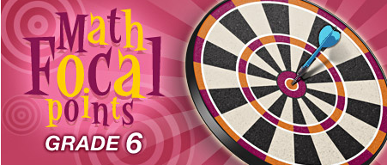### Ratio and Rate

Number and Operations: Connecting ratio and rate to multiplication and division.

Students use simple reasoning about multiplication and division to solve ratio and rate problems (e.g., “If 5 items cost \$3.75 and all items are the same price, then I can find the cost of 12 items by first dividing \$3.75 by 5 to find out how much one item costs and then multiplying the cost of a single item by 12”). By viewing equivalent ratios and rates as deriving from, and extending, pairs of rows (or columns) in the multiplication table, and by analyzing simple drawings that indicate the relative sizes of quantities, students extend whole number multiplication and division to ratios and rates. Thus, they expand the repertoire of problems that they can solve by using multiplication and division, and they build on their understanding of fractions to understand ratios. Students solve a wide variety of problems involving ratios and rates (NCTM, 2006, p.18).

Here you will find games, problems, and lesson ideas that can help middle school students extend their understanding of equivalent fractions and their mastery of whole-number multiplication and division to the concepts of ratio and rate. Communicating about Mathematics Using Games: Playing Fraction Track

Center for Digital Curriculum Research In this math game, students think about how fractions are related to a unit whole, compare fractional parts of a whole, and find equivalent fractions. Building on this experience, a teacher can help students see the connection between equivalent fractions and ratios.

Understanding Distance, Speed, and Time Relationships These two lessons are based on an online simulation of two runners along a track. Users can change the step size of each runner, control starting points, and examine a time-versus-distance graph created as the runners move. As students analyze each situation, they learn about constant rates of change and their description as formulas relating distance, speed, and time. Questions for guiding the lesson and questions for assessment are included.

Which tastes juicier? Students are challenged to decide which of four cans of grape juice concentrate requiring different amounts of water would have the strongest grape juice taste. A hint suggests forming ratios that are fractions to compare quantities. Two solutions are given, each fully illustrated with tables. Students are then offered further mixture-related questions.

Drip drops: how much water do you waste? A leaky faucet is dripping at the rate of one drop every two seconds. Students are asked to decide if the water lost in one week would fill a drinking glass, a sink, or a bathtub. The only hint is that a teaspoon holds about 20 drops. The solution demonstrates how to convert the drops to gallons using an equation or a table. Students then consider: How much water is lost in one year by a single leaky faucet? By two million leaky faucets?

How far can you go on a tank of gas? Which car will go the farthest on a single tank of gas? Students are given the mileage and gasoline tank capacity of three models of automobiles. They are encouraged to begin the problem by calculating how far each car could go in the city and on the highway. In follow-up problems, students compare the fuel efficiency of two sports cars and calculate how often a commuter would need to refuel.

Understanding Rational Numbers and Proportions To work well with ratios, learners need a solid basis in the idea of rational numbers. This complete lesson includes three well-developed activities that investigate fractions, proportion, and unit rates — all through real-world problems students encounter at a bakery.

Constant dimensions This lesson plan requires students to measure the length and width of a rectangle using both standard and nonstandard units of measure, such as pennies and beads. As students graph the ordered pairs, they discover that the ratio of length to width of a rectangle is constant, in spite of the units. This leads to the definition of a linear function and to the rule that relates the dimensions of the rectangle. An activity sheet and questions for class discussion are included.

Non-profit Tax ID # 203478467